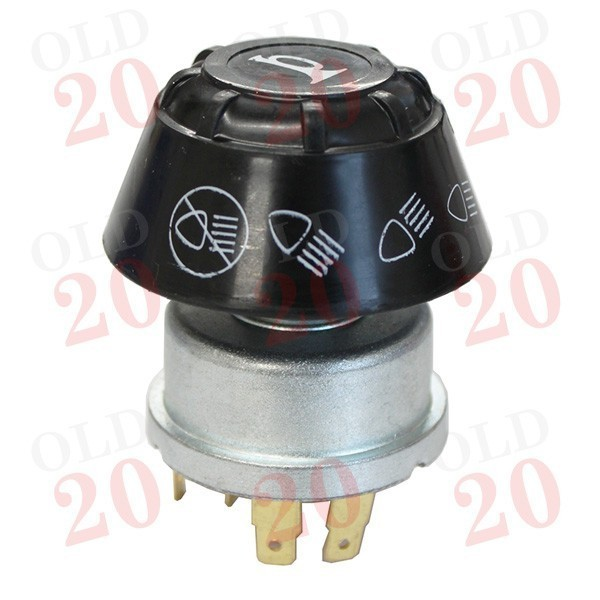View larger

# Switch - Headlight & Horn

1230107

New

Off - 1 - 2 - 3 with Horn

£ 24.00Tractors < Case IH < 84 < 684
Tractors < Case IH < 85 < 385
Tractors < Case IH < 85 < 485
Tractors < Case IH < 85 < 485XL
Tractors < Case IH < 85 < 585
Tractors < Case IH < 85 < 585XL
Tractors < Case IH < 85 < 685
Tractors < Case IH < 85 < 685XL
Tractors < Case IH < 85 < 785
Tractors < Case IH < 85 < 785XL
Tractors < Case IH < 85 < 885
Tractors < Case IH < 85 < 885XL
Tractors < Case IH < 94 < 1194
Tractors < Case IH < 94 < 1494
Tractors < Case IH < 94 < 1594
Tractors < Case IH < 94 < 1694
Tractors < David Brown < 1200 < 1210
Tractors < David Brown < 1200 < 1212
Tractors < David Brown < 94 < 1394
Tractors < David Brown < 94 < 1594
Tractors < David Brown < 94 < 1694
Tractors < Massey Ferguson < 100 < 135
Tractors < Massey Ferguson < 100 < 148
Tractors < Massey Ferguson < 100 < 154
Tractors < Massey Ferguson < 100 < 155
Tractors < Massey Ferguson < 100 < 165
Tractors < Massey Ferguson < 100 < 165 MKII
Tractors < Massey Ferguson < 100 < 168
Tractors < Massey Ferguson < 100 < 175
Tractors < Massey Ferguson < 100 < 178
Tractors < Massey Ferguson < 100 < 185
Tractors < Massey Ferguson < 100 < 188
Tractors < Massey Ferguson < 200 < 230
Tractors < Massey Ferguson < 200 < 233
Tractors < Massey Ferguson < 200 < 240
Tractors < Massey Ferguson < 200 < 245
Tractors < Massey Ferguson < 200 < 250
Tractors < Massey Ferguson < 200 < 255
Tractors < Massey Ferguson < 200 < 260
Tractors < Massey Ferguson < 200 < 261
Tractors < Massey Ferguson < 200 < 275
Tractors < Massey Ferguson < 200 < 290
Tractors < Massey Ferguson < 200 < 298
Tractors < Massey Ferguson < 500 < 550
Tractors < Massey Ferguson < 500 < 565
Tractors < Massey Ferguson < 500 < 575
Tractors < Massey Ferguson < 500 < 590
Tractors < David Brown < 90 < 1190
Tractors < David Brown < 90 < 1290
Tractors < David Brown < 90 < 1390
Tractors < David Brown < 90 < 1690

Supplier Reference :

 Section Electrical
Large round black plastic light switch with three positions. The body of the switch is metal with brass terminals. This switch can also be pushed to activate a horn (sold seperately).

Terminals: 5 (3 double, 2 single)
Positions: Off - 1 - 2 - 3
Horn: Yes